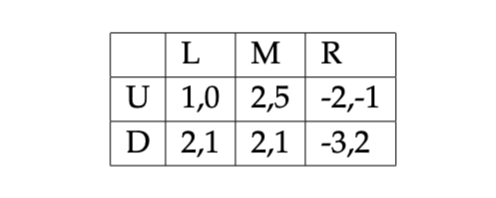# Question Question 1: Consider the situation represented by the following bimatrix: a) Is there a strictly dominant strategy equilibrium of this game? Explain. b) Is there a weakly dominant strategy equilibrium of this game? c) What are the Nash equilibria of this game? Explain using best responses. L M R U 1,0 2,5 -2,-1 D 2,1 2,1 -3,2Question 1: Consider the situation represented by the following bimatrix:a) Is there a strictly dominant strategy equilibrium of this game? Explain.

b) Is there a weakly dominant strategy equilibrium of this game?

c) What are the Nash equilibria of this game? Explain using best responses.

Transcribed Image Text: L M R U 1,0 2,5 -2,-1 D 2,1 2,1 -3,2
More
Transcribed Image Text: L M R U 1,0 2,5 -2,-1 D 2,1 2,1 -3,2